# Generalized function, derivative of a

A weak extension of the operation of ordinary differentiation. Letbe a generalized function,. The generalized (weak) derivative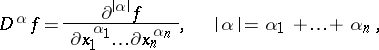of orderis defined by the equation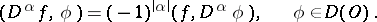(*)

Since the operation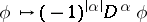is linear and continuous from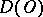into, the functional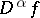defined by the right-hand side of (*) is a generalized function in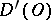. If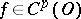, then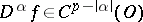for allwith.

The following properties hold for the derivatives of a generalized function: the operation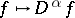is linear and continuous frominto; any generalized function inis infinitely differentiable (in the generalized sense); the result of differentiation does not depend on the order; the Leibniz formula is valid for the differentiation of a product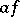, when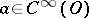; and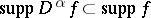.

Let. It may happen that a certain generalized derivative can be identified with some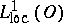-function. In this case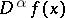is a generalized derivative of function type.

## Contents

### Examples.

1)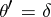, whereis the Heaviside function andis the Dirac function (cf. Delta-function for both).

2) The general solution of the equation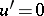in the class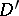is an arbitrary constant.

3) The trigonometric series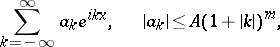converges inand it can be differentiated term-by-term ininfinitely many times.